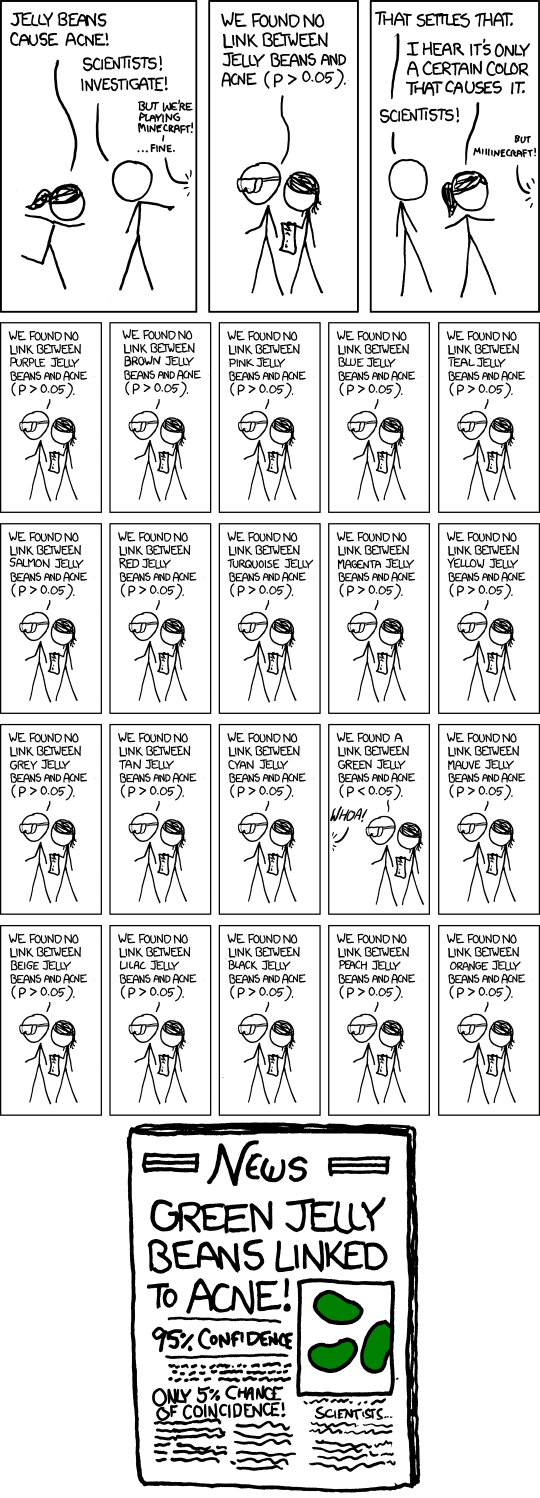# P-values are becoming embarrassing

### What is a p-value?

Anyone who has taken a basic statistics course remembers the term “p-value” and that when it’s a certain value you reject something and when it’s not you do something else. Who knows, I can’t remember. (Kidding, I teach statistics!)

Well, simply put, a p-value is the probability of getting the data you have if you assume something beforehand. Put mathematically, this is $P(\text{data}|\theta)$, where $\theta$ is some parameter you are interested in, like the mean. So let’s say we are interested in the average weight of all males at a certain company. We start by assuming it’s 185 lbs and conduct a two sided hypothesis test. Our p-value is 0.022 so we reject the assumption that the mean weight is 185. What we have actually found is $P(\text{data}|\theta=185)=0.022$. So if the real mean weight is 185 lbs, when we randomly sample a group we will get the results of our data or more extreme 2.2% of the time. Seems useful right? Maybe, maybe not. All we know is that is seems kind of unlikely to happen if the real mean weight were 185 lbs. Seems, maybe, unlikely? Ugg. Statistics deals with uncertainty but this level of uncertainty doesn’t cut it for me.

This kind of hypothesis testing tells us NOTHING about the range of the mean weight. What if we are interesting in $P(\theta=185)$? Or what if we want to know a range like $P(180 <\theta<190)$? That would be very powerful information! This probability can not be found through traditional hypothesis testing and requires uses Bayesian techniques. It has become a problem that studies find a “significant p-value” of something less than 0.05 and claim to have significant results that go against everything known about that field. Recently the American Statistical Association (AMA) commented on this problem and how this way of thinking needs to go. Hypothesis testing is a valid tool but it is much less powerful than it seems at first glance and is too often completely misunderstood.

In the war between Frequentists and Bayesian followers, it seems the AMA is siding with the latter group in this case.